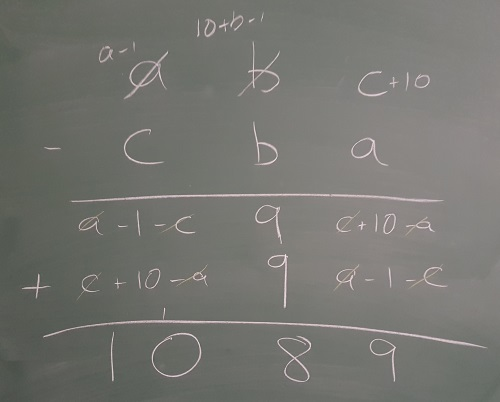#### You may also like### Double Digit### Number Detective

Follow the clues to find the mystery number.### Six Is the Sum

What do the digits in the number fifteen add up to? How many other numbers have digits with the same total but no zeros?

# Subtraction Surprise

### Why do this problem?

This problem tests students' understanding of place value and provides an opportunity for them to practise calculation methods in a context which leads to a surprising result! We hope that students will be curious about the unexpected outcome and wish to explore, and explain why it happens.

### Possible approach

"Choose a three-digit number where the first digit is bigger than the third digit. Reverse the digits, and then subtract your second number from your first one."
Give students a moment to work out the difference.
Once students have had a chance to work out the total, invite a few of them to share their answer. Enjoy the moment of surprise when everyone realises they ALL got the answer 1089.

To investigate why this happens, you may wish to show students the video from the problem.

You might like to invite them to comment on what is the same and what is different in each example. Alternatively, you could invite three students to come out to the board, and present their examples simultaneously, recreating what happens in the video.

In the video, Alison used column subtraction for all three calculations, even though there was a quicker mental method for the third one. This could provoke a useful discussion about choice of methods!

Students might use a variety of methods to perform the subtraction, and create a generalisation based on whichever method they choose (see Possible Support below for one suggestion).

If they use a column subtraction method and they are confident with representing numbers with letters, you could encourage them to use an approach like the one shown in the image below:### Key questions

What is the same in each calculation?
What is different?
Why do you always get a 9 in the tens column when you perform the subtraction?
Why do the hundreds and units of the subtraction always sum to 9?

### Possible support

Students can explain why the solution is 1089 without using column subtraction. Here is one possible explanation:

723-327 is the same as 703-307.
This is the same as 700+3-(300+7) = 700-300 +3-7
This gives 400-4, which is 4 times 99.
For any three-digit number, we will follow a similar procedure which will lead to a multiple of 99. There are only 10 of these to check, and they all give 1089.

### Possible extension

If you take the four-digit number 4321, reverse the digits, subtract, reverse the digits of the answer and add, you get 10890.
Invite students to explore whether it will work for all four-digit numbers.
If so, can they prove it? If not, can they find the conditions required to give an answer of 10890?